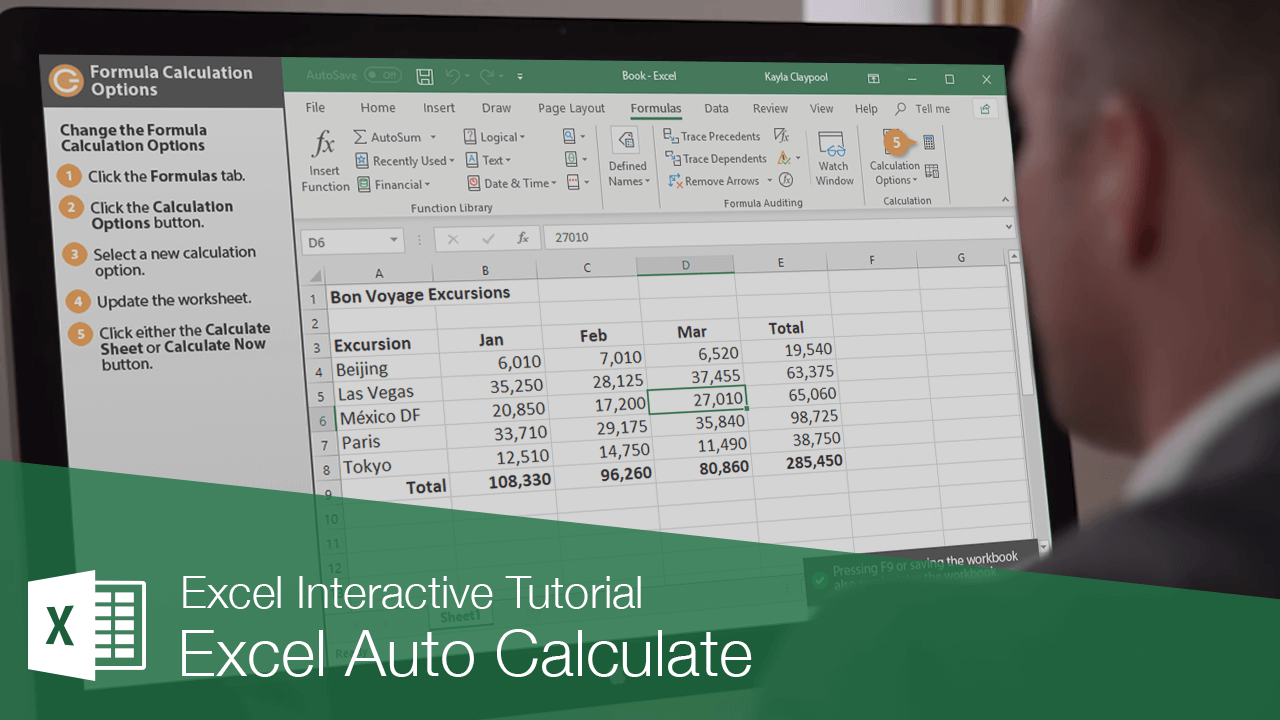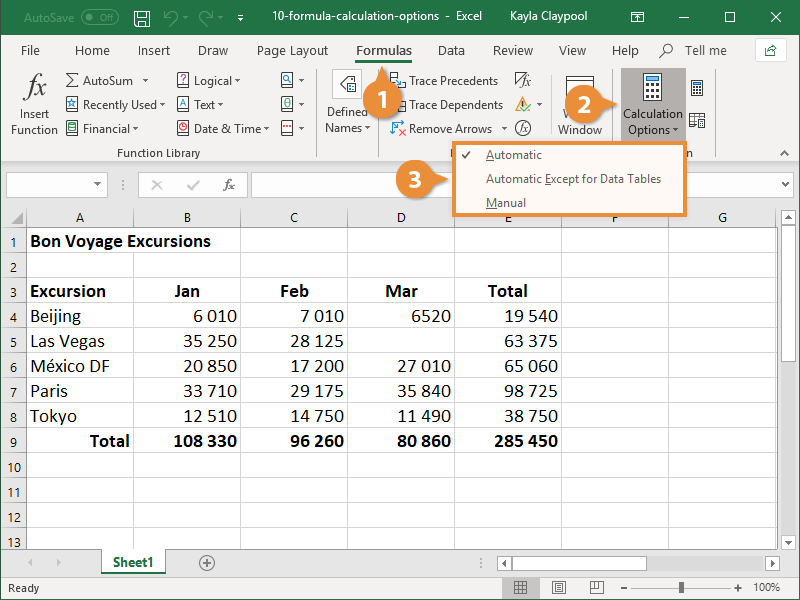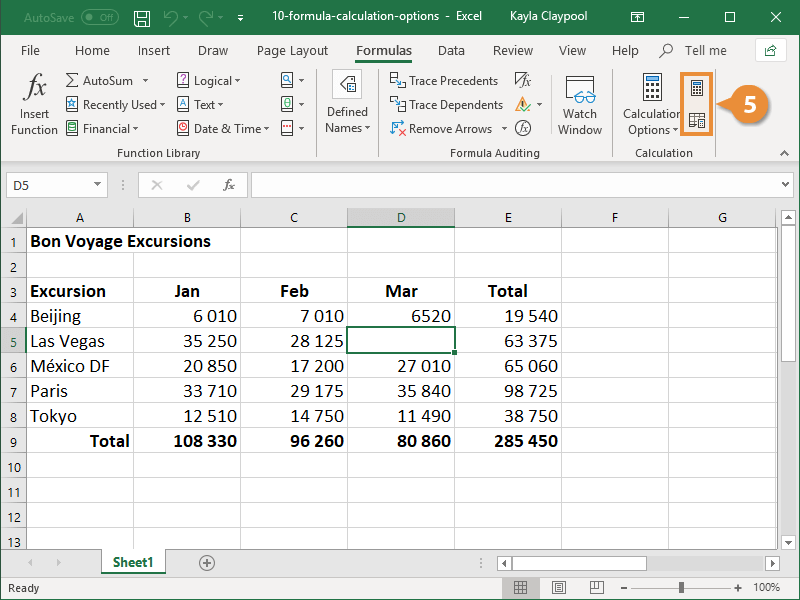Excel Auto Calculate | CustomGuide# Excel Auto Calculate

How to Change Formula Calculation Options in ExcelBy default, Excel calculates all formulas instantaneously when they are entered in a worksheet. You can, however, change the calculation options so that formulas will only calculate when directed by you. If you have large sheets of data and multiple formulas trying to calculate at once, it can take a while for all the calculations to update. If that’s the case, you’ll want to update this setting.

### Change the Formula Calculation Options

1. Click the Formulas tab.
2. Click the Calculation Options button.

Three options appear in the menu:

• Automatic: This is selected by default. Values are automatically recalculated whenever a change occurs.
• Automatic Except for Data Tables: The workbook is automatically updated with any changes. Data tables are only updated manually.
• Manual: The workbook is only updated when directed by the user.
3. Select a calculation option.4. Update the worksheet.

There are two ways to manually calculate the sheet. The Calculate Now button calculates the entire workbook, while the Calculate Sheet button only calculates the current worksheet.

5. Click either the Calculate Now or Calculate Sheet button.Pressing F9 or saving the workbook also recalculates the workbook.

The workbook or worksheet recalculates.